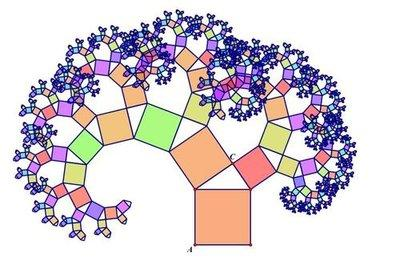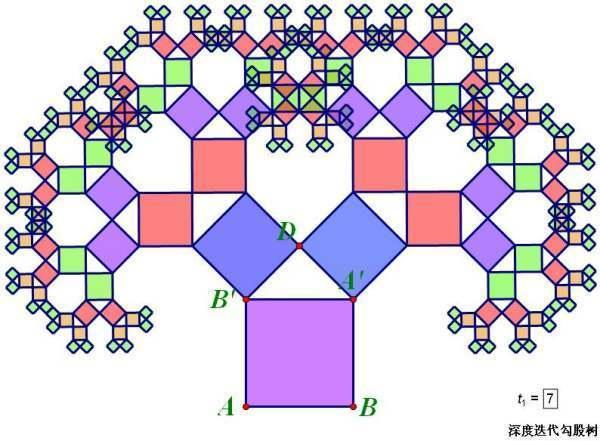##MSIPO技术圈 首页 IT技术 查看内容

# C语言实现毕达哥拉斯树 你需要知道的一切！

2023-07-13

 分形几何学是一门研究不规则几何形态的几何学学科。它涉及数学上基于递归的反馈系统，通过不断迭代的方程式生成分形。尽管分形是数学构造，但它们也存在于自然界中，并因此在艺术作品中得到应用。 计算机的出现为推动分形几何学的发展起到了重要作用。曼德尔勃罗特是一位擅长计算机和数学的法国数学家，他开创了分形几何学这一新的数学分支。分形在医学、土力学、地震学和技术分析等领域都有实际应用。 毕达哥拉斯树是根据毕达哥拉斯的勾股定理绘制的图形，它可以无限重复。因为经过多次重复后形状类似一棵树，所以被称为毕达哥拉斯树或者"勾股树"。我对解决这个问题的思路是： 1. 确定直线p1-p2，并在p1-p2的左侧找到p11-p22，使得p1-p2-p22-p11构成一个正方形。 2. 找到点p，使得p-p11-p22构成一个含有60度角的直角三角形。 3. 将直线p-p11和p-p22分别视为p1-p2，进行递归操作。递归的条件是正方形的边长大于3。 C 语言源代码如下： ``````// 定义一个结构体 Point，存储点的坐标 struct Point { double x; double y; }; // 直线的旋转（p1 是定点） Point Rotate(Point p1, Point p2, double angle) { Point r; r.x = p1.x + (p2.x - p1.x) * cos(angle) + (p2.y - p1.y) * sin(angle); r.y = p1.y + (p2.y - p1.y) * cos(angle) - (p2.x - p1.x) * sin(angle); return r; } // 直线的缩放（p1 是定点） Point Zoom(Point p1, Point p2, double ratio) { Point r; r.x = p1.x + (p2.x - p1.x) * ratio; r.y = p1.y + (p2.y - p1.y) * ratio; return r; } // 画出正方形 void Draw(Point p1, Point p2) { Point p11 = Rotate(p1, p2, 90 * PI / 180); Point p22 = Rotate(p2, p1, 270 * PI / 180);//学习交流群；558970390 POINT pts[] = { { int(p1.x + 0.5), int(p1.y + 0.5) }, { int(p2.x + 0.5), int(p2.y + 0.5) }, { int(p22.x + 0.5), int(p22.y + 0.5) }, { int(p11.x + 0.5), int(p11.y + 0.5) } }; static int color_H = 270; setfillcolor(HSVtoRGB(float(color_H), 1, 1)); setlinecolor(HSVtoRGB(float((color_H + 80) % 360), 0.5, 0.5)); color_H = (color_H + 1) % 360; fillpolygon(pts, 4); // 填充正方形颜色 if (((p22.x - p11.x) * (p22.x - p11.x) + (p22.y - p11.y) * (p22.y - p11.y)) > 3 * 3 ) { double a = 60 * PI / 180; // double a = 45 * PI / 180; Point p = Rotate(p11, p22, a); p = Zoom(p11, p, cos(a)); Draw(p, p22); Draw(p11, p); } } // 主函数 int main() { initgraph(640, 480); setbkcolor(0xfecaeb); cleardevice(); Point p1 = { 290, 400 }; Point p2 = { 350, 400 }; Draw(p1, p2); _getch(); closegraph(); return 0; }`````` 改变旋转的角度可以产生不同形状的树。例如，修改 Draw 函数里的 double a 变量为 45 度，可以得到这样的效果：